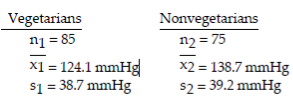• FAQ
• Contact/
/
/
Determine whether the samples independent or dependent. 21) The effectiveness of
Not my Question
Flag Content

# Question : Determine whether the samples independent or dependent. 21) The effectiveness of : 1728905

Determine whether the samples are independent or dependent.

21) The effectiveness of a headache medicine is tested by measuring the intensity of a headache in patients before and after drug treatment. The data consist of before and after intensities for each patient.

A) Independent samples B) Dependent samples

Use the traditional method to test the given hypothesis. Assume that the samples are independent and that they have been randomly selected

22) Use the given sample data to test the claim that p1 < p2. Use a significance level of 0.10.

Sample : n1 = 462 x1 = 84

Sample 2: n2 = 380 x2 = 95

Assume that you want to test the claim that the paired sample data come from a population for which the mean difference is μd = 0. Compute the value of the t test statistic. Round intermediate calculations to four decimal places as needed and final answers to three decimal places as needed.

23)

x 28 31 20 25 28 27 33 35

---------------------------------

y 26 27 26 25 29 32 33 34

A) t = -1.480 B) t = -0.185 C) t = -0.523 D) t = 0.690

Test the indicated claim about the means of two populations. Assume that the two samples are independent simple random samples selected from normally distributed populations. Do not assume that the population standard deviations are equal. Use the traditional method or P-value method as indicated.

24) A researcher was interested in comparing the amount of time (in hours) spent watching television by women and by men. Independent simple random samples of 14 women and 17 men were selected, and each person was asked how many hours he or she had watched television during the previous week. The summary statistics are as follows.

Women Men

x1 = 12.5 hr x2 = 13.8 hr s1 = 3.9 hr s2 = 5.2 hr

n1 = 14 n2 = 17

Use a 0.05 significance level to test the claim that the mean amount of time spent watching television by women is smaller than the mean amount of time spent watching television by men. Use the traditional method of hypothesis testing.

25) A researcher wishes to determine whether the blood pressure of vegetarians is, on

average, lower than the blood pressure of nonvegetarians. Independent simple random samples of 85 vegetarians and 75 nonvegetarians yielded the following sample statistics for systolic blood pressure:Use a significance level of 0.01 to test the claim that the mean systolic blood pressure of vegetarians is lower than the mean systolic blood pressure of nonvegetarians. Use the P-value method of hypothesis testing.

## Solution 5 (1 Ratings )

Solved
Statistics 8 Months Ago 46 Views